# Finding the Domain & Range of Functions with Inequalities

An error occurred trying to load this video.

Try refreshing the page, or contact customer support.

Coming up next: How to Graph an Absolute Value and Do Transformations

### You're on a roll. Keep up the good work!

Replay
Your next lesson will play in 10 seconds
• 0:03 Domain & Range of…
• 3:50 Absolute Value
• 5:16 Polynomial Inequalities
• 6:36 Steps to Find the Domain
• 12:41 Lesson Summary
Save Save

Want to watch this again later?

Timeline
Autoplay
Autoplay
Speed Speed Audio mode

#### Recommended Lessons and Courses for You

Lesson Transcript
Instructor
Anna Teper-Dillard
Expert Contributor
Will Welch

Will has a doctorate in chemistry from the University of Wyoming and has experience in a broad selection of chemical disciplines and college-level teaching.

In this lesson, you will learn what domain and range are and how to find them in absolute value and polynomial functions with inequalities. You will look at domain and range expressed as intervals, inequalities, and shaded graphs.

## Domain and Range of Linear Inequalities

Domain is the set of all x values, the independent quantity, for which the function f(x) exists or is defined. For example, if we take the linear function:

f(x) = 2x + 3, we can evaluate f(x) at any point, and we will get a real answer for y: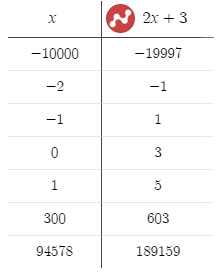Thus, the domain of f(x) is all real numbers, or negative infinity to infinity.

Range is the set of all y values, the dependent quantity, that will result from substituting all x values (the domain) into the function.

So the range of f(x) = 2x + 3 is also all real numbers, because no matter what value of x is, we can always multiply that number by 2 and add 3.

That was an equation, but how will an inequality change the domain and range of this linear function? Well, we'll see that the inequality does not affect the domain and range of linear functions at all. Let's not confuse domain and range with a solution to an inequality. These two concepts are different. Domain and range mean all possible values of x and y that could be substituted to the inequality. A solution means all possible values that make the inequality statement true.

Let's look at an example:

f(x) > 2x + 3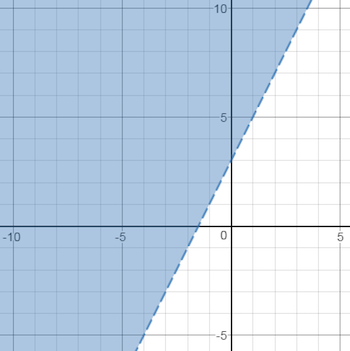The domain is still all real numbers, because the inequality sign changes the relationship between the y and the x, and not the actual set of values we could substitute for either variable. The solution to the inequality states that the y values have to be greater than 2x + 3, not equal. So while the range is still all real numbers, the solution is the shaded area, for which y will always be greater than the line of the function. Let's check a couple of examples: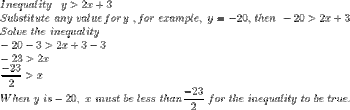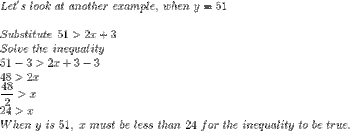As we see, whatever y value we picked, there will always be an x value that will make the inequality true. Therefore, domain and range of linear inequalities will always be all real numbers, which again, is not the same as the solution to the inequality.

### Absolute Value

Absolute value is the distance from zero, regardless of the direction. Therefore, the absolute value is always positive. The absolute value function uses the absolute value symbol (two parallel lines) to express only positive output for either positive or negative input.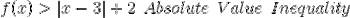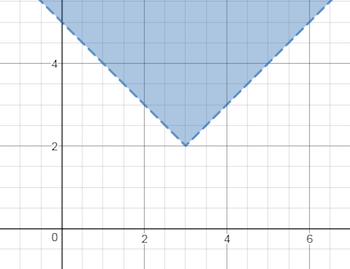The domain is all real numbers, because an absolute value is still a linear function. The range, however, will depend on the vertex of the absolute value function (the minimum or the maximum). The vertex of an absolute value function (and quadratic as well) is the lowest or the highest possible y value. With this particular inequality, the function has a minimum, and the range is all y values greater than the minimum.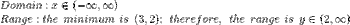Let's look at another example: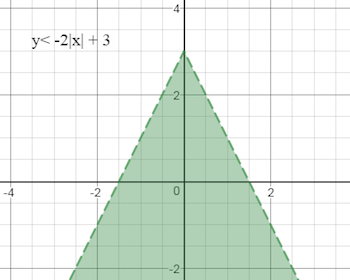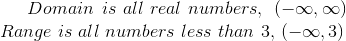## Polynomial Inequalities

A polynomial function is a combination of coefficients, variables and exponents, where the exponent has to be a positive integer (a whole number). For example, the basic quadratic function is a polynomial f(x) = x2.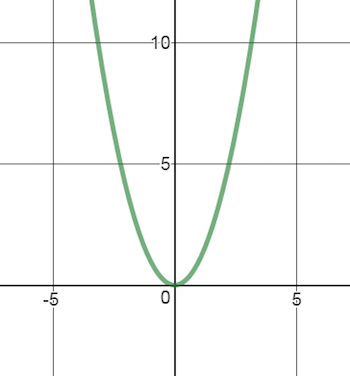To unlock this lesson you must be a Study.com Member.

## Writing and Graphing Real Life Inequalities

The following exercise is meant to help students identify how an inequality can represent a real life situation and provide an intuitive way of thinking about their domains and ranges.

### Writing Inequalities

Come up with inequalities to express the following relationships.

1. You have $50 to spend on a taxi. Taxi rates vary, so the distance you can travel depends on the rate they charge. 2. I am building a rectangular fence and I have 100 yards of fencing. There is a maximum area that can be enclosed this way. (Hint: It might help to find the formula for the area and graph that before writing the inequality for this one.) ### Graphing Inequalities Graph each inequality described in the above section and identify the domain and range for each one. #### Solutions 1. The taxi rate per mile multiplied by the distance you travel must be less than or equal to$50, or d * r <= 50.

Written in terms of a dependent and independent variable, d<= 50/r.

Plotting y <= 50/x :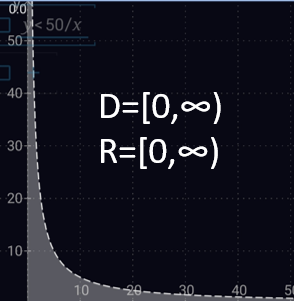Since we technically are given no lower limit on rates, the domain goes up to infinity. The range is the same.

If the lowest rate was, for example 50 cents per mile, the domain would have a lower limit of \$0.50 and the range would have an upper limit of 100 miles.

1. This requires a little bit of algebra. First, use the fact that a rectangle has two sides of equal width and two sides of equal length. Therefore, you have 100 yards.

2W + 2L = 100

Rewrite in terms of one of the sides:

W = (100 - 2L)/2

Then, the area of the rectangle is:

A=L(100 - 2L)/2 = -L^2 + 50L

From the graph of this, we can see that this is a parabola with a vertex at (25, 625).

This means that maximum area possible is 625 square yards so an inequality for situation could be:

L(100 - 2L)/2 <= 625. or -L^2 + 50L <= 625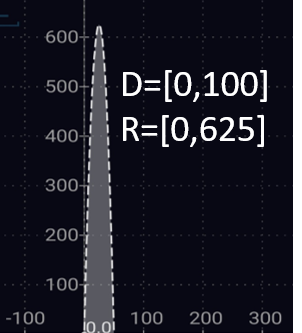Notice that the domain only goes up to 100 yards? Why? Because there have to be 2 long sides of a rectangle, so even if the width was somehow zero, each long side would be 100.

### Register to view this lesson

Are you a student or a teacher?

#### See for yourself why 30 million people use Study.com

##### Become a Study.com member and start learning now.
Back
What teachers are saying about Study.com

### Earning College Credit

Did you know… We have over 200 college courses that prepare you to earn credit by exam that is accepted by over 1,500 colleges and universities. You can test out of the first two years of college and save thousands off your degree. Anyone can earn credit-by-exam regardless of age or education level.